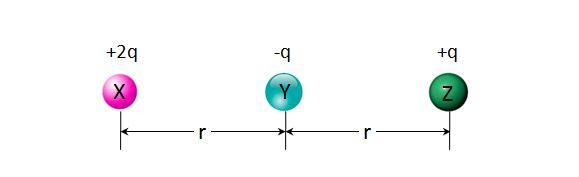# Which one is stronger?The above are $3$ charged metal balls X, Y and Z separated by an equal distance $r$ on a straight line. Let $\lvert{F_Y}\rvert$ be the magnitude of the electrostatic force on Y, and let $\lvert{F_Z}\rvert$ be the magnitude of the electrostatic force on Z. What is the ratio $\lvert{F_Y}\rvert : \lvert{F_Z}\rvert?$

×

Problem Loading...

Note Loading...

Set Loading...